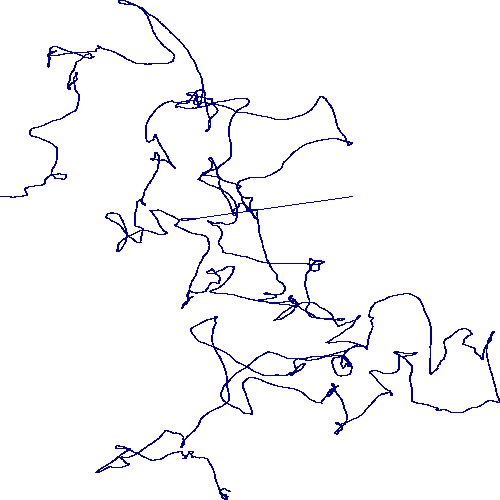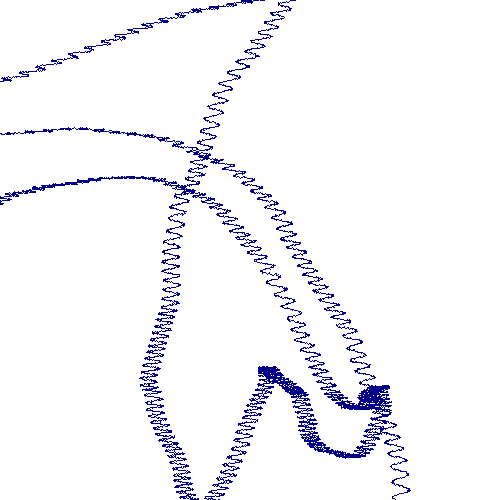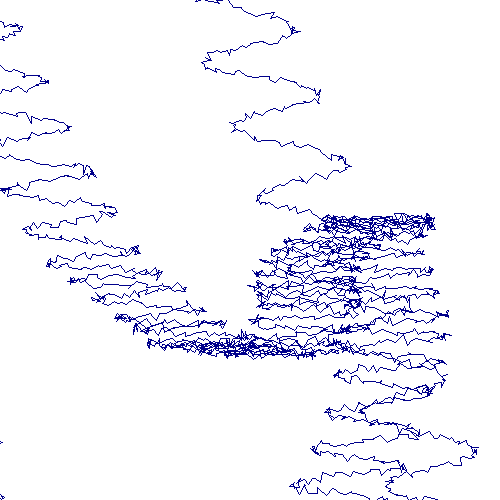# Trajectories#

With GPS-enabled devices, it’s easy to collect a large quantity of trajectory data, i.e. a connected series of points in 2D or 3D. However, it’s not so easy to plot large datasets with most plotting programs, and so people generally downsample the trajectories, which can hide important features of the data. Here we show how to use datashader to look at all the datapoints even for large datasets, faithfully displaying the data at the highest level, while revealing additional structure when examining small regions of the data.

```import pandas as pd
import numpy as np
```

## Create some fake data#

Here we create a fake trajectory in a 2D space by calculating a random walk with momentum and then adding various perturbations:

1. a sine-based displacement of the x axis to simulate e.g. a mechanical problem

2. random noise on both x and y to simulate measurement uncertainty

3. a completely arbitrary bogus value at a fixed location, to simulate corrupted data

```# Constants
np.random.seed(1)
n = 1000000 # Number of points
f = filter_width = 5000 # momentum or smoothing parameter, for a moving average filter

# filtered random walk
xs = np.convolve(np.random.normal(0, 0.1, size=n), np.ones(f)/f).cumsum()
ys = np.convolve(np.random.normal(0, 0.1, size=n), np.ones(f)/f).cumsum()

# Add "mechanical" wobble on the x axis
xs += 0.1*np.sin(0.1*np.array(range(n-1+f)))

xs += np.random.normal(0, 0.005, size=n-1+f)
ys += np.random.normal(0, 0.005, size=n-1+f)

# Add a completely incorrect value
xs[int(len(xs)/2)] = 100
ys[int(len(xs)/2)] = 0

# Create a dataframe
df = pd.DataFrame(dict(x=xs,y=ys))

# Default plot ranges:
x_range = (xs.min(), xs.max())
y_range = (ys.min(), ys.max())

df.tail()
```
x y
1004994 65.164829 -105.064056
1004995 65.177603 -105.069781
1004996 65.190898 -105.071699
1004997 65.194054 -105.054657
1004998 65.204752 -105.073366

## Static Plots#

With datashader, it’s quick and easy to plot the entire 1-million-point dataset, without any downsampling:

```def create_image(x_range=x_range, y_range=y_range, w=500, h=500):
cvs = ds.Canvas(x_range=x_range, y_range=y_range, plot_height=h, plot_width=w)
agg = cvs.line(df, 'x', 'y', agg=ds.any())
```
```%time create_image()
```
```CPU times: user 2.61 s, sys: 35.8 ms, total: 2.65 s
Wall time: 2.65 s
```Here you can immediately see the long straight line that results from the one stray value. A single stray such value is very unlikely to be discovered in any downsampled plot, but is obvious here.

Similarly, the plot above suggests that the trajectory is relatively smooth, but if you zoom in further you can see the wobble that’s not obvious at the high-level view:

```create_image(x_range=(95.0,101.0), y_range=(-61,-55))
```Zooming in even further reveals the low-level noise displacing around each datapoint:

```create_image(x_range=(98.8,99.8), y_range=(-60.2,-59.2))
```Downsampling the dataset would cover up each of these problems. If such data were then used for e.g. calculating total trajectory length, the result would be wildly incorrect – the single incorrect reading would greatly increase the calculated length, while downsampling the “measurement noise” and the added wobble would decrease the reported length. Datashading makes the full dataset available for visual inspection, so that issues like this can be detected rather than being covered up by downsampling.

## Dynamic Plots#

Specifying hard-coded ranges as above is awkward, so it’s much more natural to simply zoom in interactively, which can be done using the `datashade` operation imported from HoloViews.

```from holoviews.operation.datashader import datashade, spread
import holoviews as hv
hv.extension('bokeh')
```Now it is possible to build an interactive visualization by passing HoloViews `Path` object to the `datashade` operation:

```opts = hv.opts.RGB(width=900, height=500, aspect='equal')